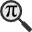## General

Display information for equation id:math.225368.4 on revision:225368

* Page found: Error function (eq math.225368.4)

(force rerendering)

Cannot find the equation data in the database. Fetching from revision text.

Occurrences on the following pages:

Hash: 2f51310acab41649af988ccebfe4186d

TeX (original user input):

\Phi


TeX (checked):

\Phi


### LaTeXML (experimental; uses MathML) rendering

MathML (497 B / 259 B) :${\displaystyle\Phi}$
<math xmlns="http://www.w3.org/1998/Math/MathML" id="p1.1.m1.1" class="ltx_Math" alttext="{\displaystyle\Phi}" display="inline">
<semantics id="p1.1.m1.1a">
<mi mathvariant="normal" id="p1.1.m1.1.1" xref="p1.1.m1.1.1.cmml">Φ</mi>
<annotation-xml encoding="MathML-Content" id="p1.1.m1.1b">
<ci id="p1.1.m1.1.1.cmml" xref="p1.1.m1.1.1">Φ</ci>
</annotation-xml>
<annotation encoding="application/x-tex" id="p1.1.m1.1c">{\displaystyle\Phi}</annotation>
</semantics>
[/itex]


SVG (1.292 KB / 768 B) :

### MathML with SVG or PNG fallback (recommended for modern browsers and accessibility tools) rendering

SVG image empty. Force Re-Rendering

SVG (0 B / 8 B) :

PNG (0 B / 8 B) :

$\Phi$## Translations to Computer Algebra Systems

### Translation to Maple

In Maple: Phi

\Phi: Could be the golden ratio conjugate.

But this system don't know how to translate it as a constant. It was translated as a general letter.

### Translation to Mathematica

In Mathematica: \[CapitalPhi]

\Phi: Could be the golden ratio conjugate.

But this system don't know how to translate it as a constant. It was translated as a general letter.

## Similar pages

Calculated based on the variables occurring on the entire Error function page

## Identifiers

• $\Phi$### MathML observations

0results

0results

no statistics present please run the maintenance script ExtractFeatures.php

0 results

0 results